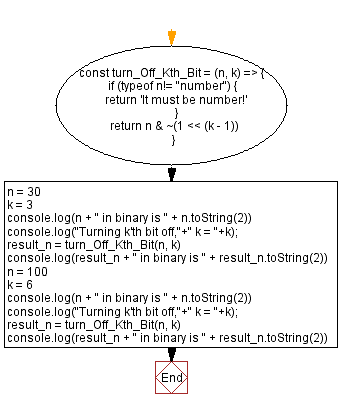# JavaScript - Turn off the kth bit of a given number

## JavaScript Bit Manipulation: Exercise-9 with Solution

Write a JavaScript program to turn off the kth bit in a given number. Return the updated number.

Test Data:
(30, 3)-> 26

Explanation:
Binary format of 30 is -> 11110
Fortmat of the said number after off the 3rd bit -> 11010
After converting 11010 to decimal : 11010 -> 26
(100, 6) -> 68
Explanation:
Binary format of 100 is -> 1100100
Fortmat of the said number after off the 6th bit -> 1000100
After converting 1000100 to decimal : 1000100 -> 68

Sample Solution:

JavaScript Code:

``````const turn_Off_Kth_Bit = (n, k) => {
if (typeof n!= "number") {
return 'It must be number!'
}
return n & ~(1 << (k - 1))
}
n = 30
k = 3
console.log(n + " in binary is " + n.toString(2))
console.log("Turning k'th bit off,"+" k = "+k);
result_n = turn_Off_Kth_Bit(n, k)
console.log(result_n + " in binary is " + result_n.toString(2))
n = 100
k = 6
console.log(n + " in binary is " + n.toString(2))
console.log("Turning k'th bit off,"+" k = "+k);
result_n = turn_Off_Kth_Bit(n, k)
console.log(result_n + " in binary is " + result_n.toString(2))
```
```

Sample Output:

```30 in binary is 11110
Turning k'th bit off, k = 3
26 in binary is 11010
100 in binary is 1100100
Turning k'th bit off, k = 6
68 in binary is 1000100
```

Flowchart:Live Demo:

See the Pen javascript-bit-manipulation-exercise-9 by w3resource (@w3resource) on CodePen.

* To run the code mouse over on Result panel and click on 'RERUN' button.*

Improve this sample solution and post your code through Disqus

What is the difficulty level of this exercise?

Test your Programming skills with w3resource's quiz.

﻿